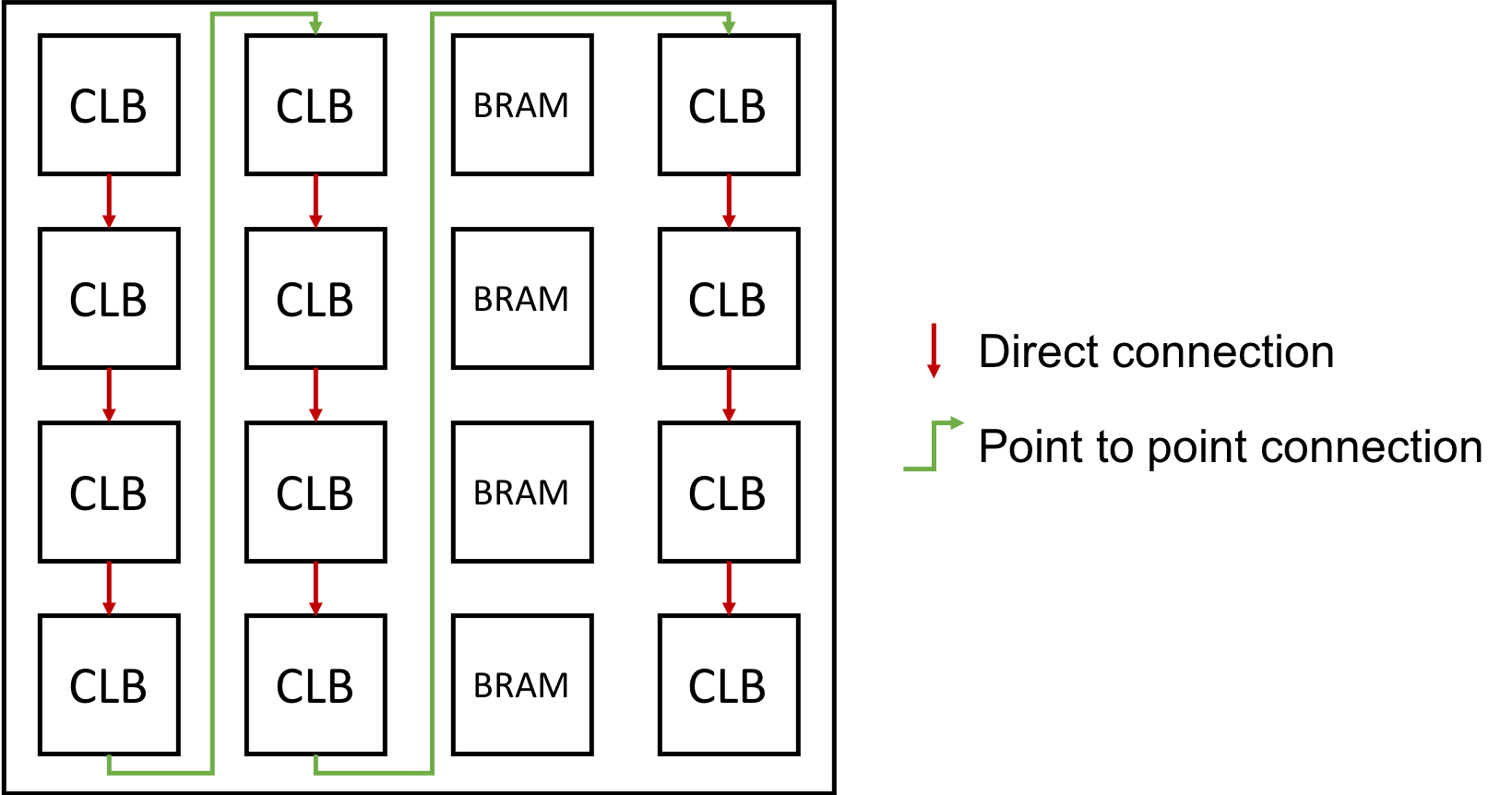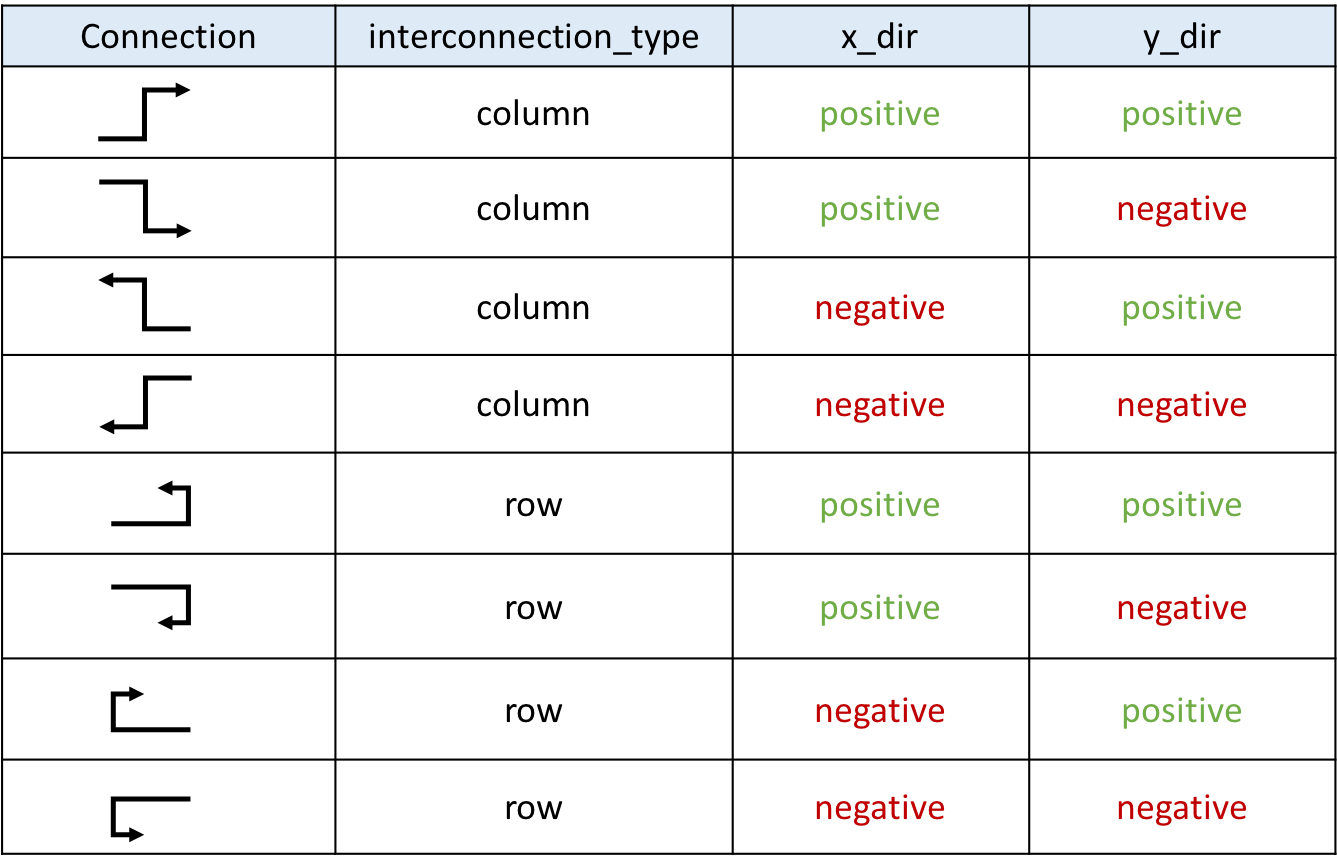# Inter-Tile Direct Interconnection extensions¶

This section introduces extensions on the architecture description file about existing interconnection description.

## Directlist¶

The original direct connections in the directlist section are documented here. Its description is given below:

```<directlist>
<direct name="string" from_pin="string" to_pin="string" x_offset="int" y_offset="int" z_offset="int" switch_name="string"/>
</directlist>
```

Note

These options are required

Our extension include three more options:

```<directlist>
<direct name="string" from_pin="string" to_pin="string" x_offset="int" y_offset="int" z_offset="int" switch_name="string" interconnection_type="string" x_dir="string" y_dir="string"/>
</directlist>
```

Note

these options are optional. However, if interconnection_type is set x_dir and y_dir are required.

interconnection_type="<string>"

the type of interconnection should be a string. Available types are `NONE` | `column` | `row`, specifies if it applies on a column or a row ot if it doesn’t apply.

x_dir="<string>"

Available directionalities are `positive` | `negative`, specifies if the next cell to connect has a bigger or lower `x` value. Considering a coordinate system where (0,0) is the origin at the bottom left and `x` and `y` are positives:

• x_dir=”positive”:

• interconnection_type=”column”: a column will be connected to a column on the `right`, if it exists.

• interconnection_type=”row”: the most on the `right` cell from a row connection will connect the most on the `left` cell of next row, if it exists.

• x_dir=”negative”:

• interconnection_type=”column”: a column will be connected to a column on the `left`, if it exists.

• interconnection_type=”row”: the most on the `left` cell from a row connection will connect the most on the `right` cell of next row, if it exists.

y_dir="<string>"

Available directionalities are `positive` | `negative`, specifies if the next cell to connect has a bigger or lower x value. Considering a coordinate system where (0,0) is the origin at the bottom left and x and y are positives:

• y_dir=”positive”:

• interconnection_type=”column”: the `bottom` cell of a column will be connected to the next column `top` cell, if it exists.

• interconnection_type=”row”: a row will be connected on an `above` row, if it exists.

• y_dir=”negative”:

• interconnection_type=”column”: the `top` cell of a column will be connected to the next column `bottom` cell, if it exists.

• interconnection_type=”row”: a row will be connected on a row `below`, if it exists.

## Example¶

For this example, we will study a scan-chain implementation. The description could be:

```<directlist>
<direct name="scff_chain" from_pin="clb.sc_out" to_pin="clb.sc_in" x_offset="0" y_offset="-1" z_offset="0" interconnection_type="column" x_dir="positive" y_dir="positive"/>
</directlist>
```

Fig. 24 is the graphical representation of the above scan-chain description on a 4x4 FPGA.Fig. 24 An example of scan-chain implementation¶

In this figure, the red arrows represent the initial direct connection. The green arrows represent the point to point connection to connect all the columns of CLB.

## Truth table¶

A point to point connection can be applied in different ways than showed in the example section. To help the designer implement his point to point connection, a truth table with our new parameters id provided below.

Fig. 25 provides all possible variable combination and the connection it will generate.Fig. 25 Point to point truth table¶